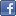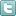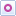EE Times-India > EDA/IP

EDA/IP# Software PLL syncs to line using filter

Posted: 20 Oct 2015Print Version

Keywords:software phase-locked loop  SPLL  ECG  DSP

A software phase-locked loop (SPLL) is employed to generate a synchronous reference to common-mode powerline interference in two-electrode ECG amplification. Though intended for ECG signal processing, it can easily be adapted to various DSP applications where frequency synchronisation is a must.

The basic SPLL structure consists of three blocks: Phase detector (PHD), Loop filter (LF), and Digitally-controlled oscillator (DCO) (figure 1). The input signal Vin is processed in digital form: the PHD is a multiplier – its output, the product of two signals: the input sine wave (fin), and the DCO sine output (fref). Sine wave mixing is preferable when low jitter is a must.Figure 1: Software PLL structure.

The LF integrates the PHD output data in time and increases the resolution due to averaging, so the m-bit wide DCO input can be larger than the n-bit wide signals.

The DCO operates as a digital-to-frequency converter with sine output, and must be able to match the expected input frequency range.

The key part of the SPLL is the loop filter. It must be carefully designed to provide stable system response with appropriate settling time.

Loop gain analysis and design methodology of the SPLL is given in , where it is shown how the SPLL z-domain transfer function can be derived from its analogue s-domain prototype using backward difference s-plane to z-plane mapping.

The SPLL control loop consists of two integrators: one is hidden in the DCO, and another one is in the LF. Because the LF integrator serves a second integrator in the loop, it must be bypassed with a forward path to maintain stability, as seen in figure 2. The disadvantage of this topology is that the forward path increases the remaining ripple at the DCO input, which is converted to jitter at the DCO output. The problem can be overcome with a comb filter with notches at all powerline harmonics. The simplest comb filter rejecting all harmonics is a one-period moving-average filter (averager) . Adding this to the loop greatly reduces the remaining ripple at the DCO input, and this is the heart of the Design Idea.Figure 2: Loop filter structure.

The LF transfer function is given with Eq. (1), where the first multiplicand is the transfer function of the averager, and the second multiplicand is the transfer function of the bypassed integrator:T is the sampling period: T=1/fs. TPL is the powerline period: TPL=1/fPL. ki and kz are the gain coefficients in the integrator and in the forward paths. For sampling rate fs=2kHz or T=0.5ms, fPL=50Hz (TPL=20ms), ki=1/128≈0.0078, and kz=8, Eq. (1) can be rewritten as Eq. (2):The LF transfer function, given with Eq. (1), can be realised with the signal flow schematic shown in figure 2.

The SPLL is implemented and tested on the STM32F407 microcontroller, running at fclk=100MHz. The microcontroller incorporates a 12bit ADC which is used to convert the input signal at sampling rate fs=2kHz. One LSb corresponds to 3V/4096=0.732mV. The DCO range is ±2Hz. It is controlled with a 12bit word; thus, the DCO sensitivity is 1mHz/LSb, or 1.36Hz/V. To avoid floating point multiplications, the DCO generates a 256-level sine wave. The mixer output is divided by 256 to set the correct loop gain. To minimise the DCO's remaining ripple, the ADC sampling rate is a multiple of the generated frequency fref. Thus, the averager, included in the LF, is maximally effective in rejection the powerline harmonics.

Comment on "Software PLL syncs to line using fil..."

Top Ranked Articles

WebinarsVisit Asia Webinars to learn about the latest in technology and get practical design tips.

Search EE Times India
Services

Go to topConnect on FacebookFollow us on TwitterFollow us on Orkut
﻿